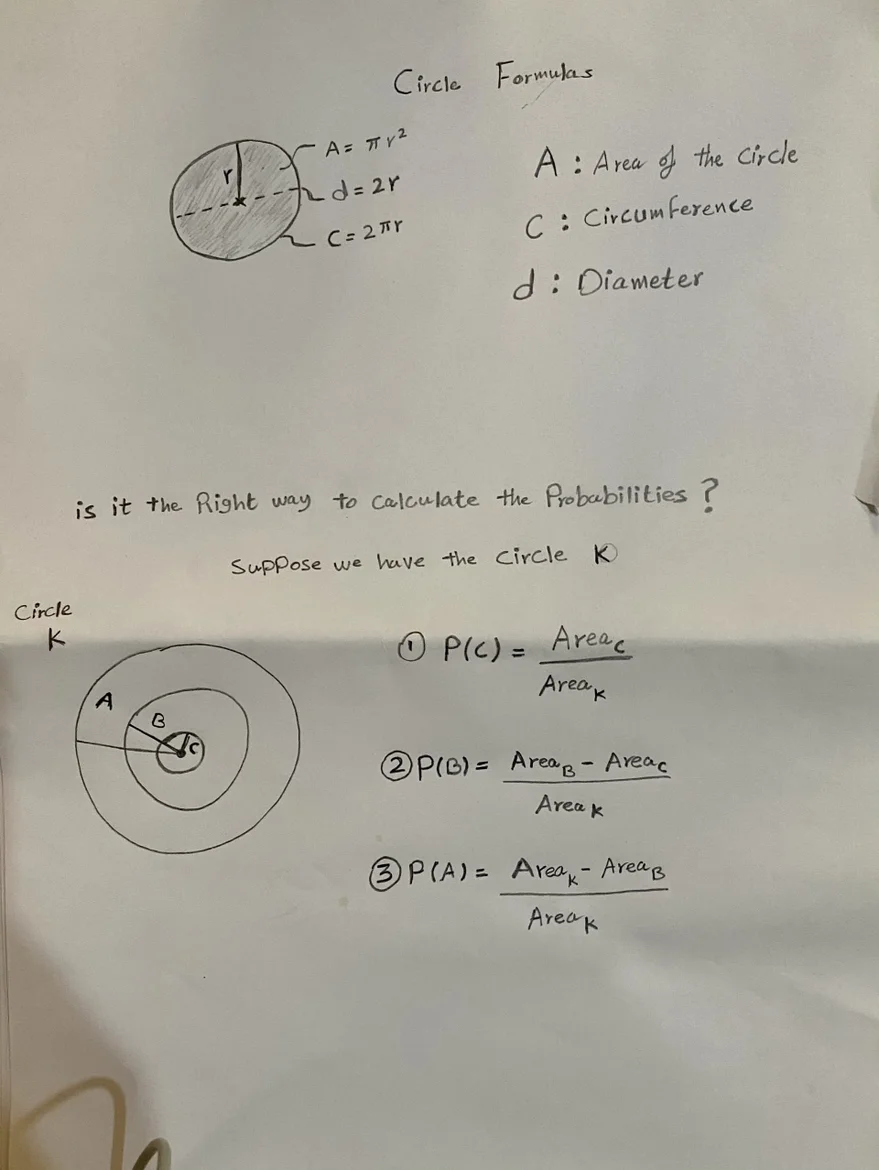16 Jun 2023

Posted on:

08 Jun 2023

1

# hello, is this correct?

A= pi * r^2

Circumferance= 2 * pie * r

d = 2r

if we have got the information about the circle is this right?

what is the likelihood of hitting a point inside circle C which is inside cirlce K ?

is the area of circle C divided by the area of circle K

what is the likelihood of hitting a point inside the donut circle B which is inside K

Area of  B - Area of C / Area of Circle K

hitting a point inside A

p(A) =  1- (p(C) + p(B))

or

100% - ( (p(C) + p(B))

or

Area of K - Area of B / Area kInstructor
Posted on:

16 Jun 2023

1

Hey Feras,

Thank you for reaching out!

So long as you assume that each point on the board is equally likely to be hit, your solution to the problem is correct :)

Kind regards,

365 Hristina